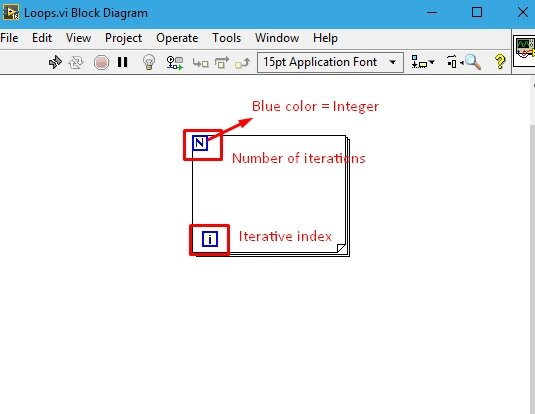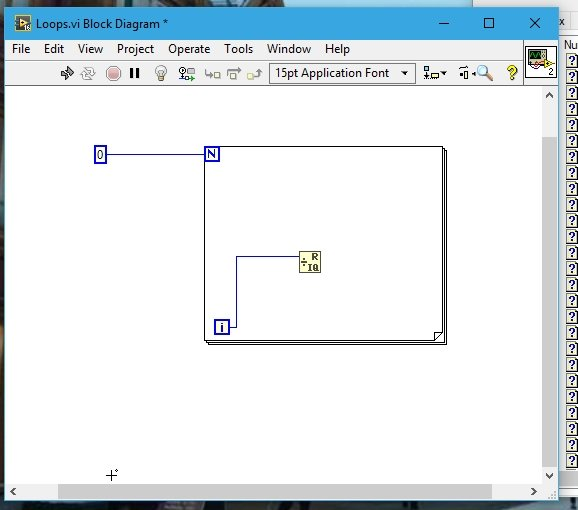# How use to loops in labview : tutorial 3

In this tutorial you will learn the use of loops. You can use any (for or while) loop after going through this tutorial and doing the tasks assigned. Step by step elaboration of the program taken as example is given in the programming section and at the end of the tutorial I have given an assignment exercise which is compulsory for the reader to do, If he/she is willing to actually learn programming skills in LabView.

Introduction to loops in labview

Loops are used to run a specific part of a code iteratively. If you are a user of any programming language you must be familiar with loops. Some of the most commonly used loops in every programming language are for loop, while loop and do….while loops. Loops are mostly used in the programs where we need the result of a function at various data points. We can update the value of variable, store values in arrays (we will discuss them in upcoming tutorials) and also plot graphs (we will discuss plotting in upcoming tutorials) and many more things iteratively.  In programming language, a loop is defined as sequence of commands which are required to be repeated until a certain condition provided by the programmer is satisfied. After the condition is satisfied the control flow leaves the loop and stop executing the program over and over again.

Typically, for loop is used when the number of iterations are known and while loop allows the code to be executed repeatedly depending upon a Boolean condition. If the condition is true the code inside the loop is executed and if it is false the loop is skipped. While working with loops, one must remember that the condition given to the loop must converge to satisfaction, i.e. the if the condition is never going to be satisfied ever, your loop will run infinitely, and ultimately you program will never stop, which is not a good programming skill.

### Programs using loop in labview

Figure 1: Saving a VI

Figure 2: Placement of for loops

• In the function block of for loop you can see two small blue boxes, one with an ‘i’ in it and the other with ‘N’. The color of the boxes are very important, they represents the data type of the variables. We will study the data types of variables in detail in upcoming tutorial. The blue color here represents the integer data type of the variable. Coming back to the N and i variables, ‘N’ variable represents the number of iterations and ‘i’ is the iterative index. As shown in the figure below.Figure 3: For loop

### Loops examples in labview

• Now let’s do some programming to see the functionality of a ‘for’ loop. From the function palette select Numeric and then select Quotient and remainder and place it inside the block.
• Always place the block inside the for loop if you want it to be processed iteratively, and if the block is just providing input to the loop then place it outside the loop and the VI will automatically provide you with a knob to attach it to the loop.
• Connect the upper terminal of the quotient and remainders block to the iterative index ‘i’ variable. Now, place a constant value outside the loop and connect it to the loop through N (the constant will provide value to the N (number of iterations) variable.Figure 4: Placement of blocks and constants

Figure 5: Not placement

Figure 6: Test light

Figure 7: Placing wires.

Figure 8: Wait block placement

Figure 9: Complete block diagram

Figure 10: With i as a multiple of 5

Figure 11: With i not a multiple of 5

The program clarifies the use of for loop. If you are an early programmer you might be thinking why the LED blinks at multiples of 5. The output of the Quotient and remainder function block given the remainder as well as the quotient when the two input numbers are divided. We connect an equal to 0? Block at the remainder of this block. The remainder of this block will be 0 when the value of i is a multiple of 5, and a number otherwise. The equal to 0? Block compares the input value to 0. If the input to this block is 0 it gives a logical 1 as output and if the input is not equal to zero the output is a logical 0. LED indicator is a Boolean indicator. It blinks when the input to it is Boolean 1 and remains off if the input is 0. Summing this up in a nut shell shows that when i is a multiple of 5 the remainder of I divided by 5 will be zero which when compared with 0 in equal to 0 comparator gives a Boolean 1 which when fed to LED, turns the LED on.

### Exercise for loops in labview

• Do the above task using while loop.

(Hint: Instead of N (number of iterations) while loop contains a condition which when satisfied will stop executing the loop)